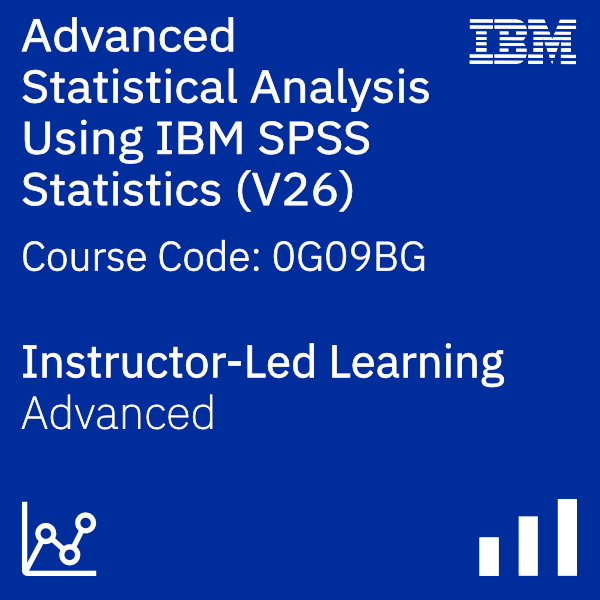# Overview

This course provides an application-oriented introduction to advanced statistical methods available in IBM SPSS Statistics. Students will review a variety of advanced statistical techniques and discuss situations in which each technique would be used, the assumptions made by each method, how to set up the analysis, and how to interpret the results. This includes a broad range of techniques for predicting variables, as well as methods to cluster variables and cases.

# Audience

IBM SPS Statistics users who want to learn advanced statistical methods to be able to better answer research questions.

# Prerequisites

• Experience with IBM SPSS Statistics (version 18 or later)
• Knowledge of statistics, either by on the job experience, intermediate-level statistics oriented courses, or completion of the Statistical Analysis Using IBM SPSS Statistics (V26) course.

# Objective

• Introduction to advanced statistical analysis
• Grouping variables with Factor Analysis and Principal Components Analysis
• Grouping cases with Cluster Analysis
• Predicting categorical targets with Nearest Neighbor Analysis
• Predicting categorical targets with Discriminant Analysis
• Predicting categorical targets with Logistic Regression
• Predicting categorical targets with Decision Trees
• Introduction to Survival Analysis
• Introduction to Generalized Linear Models
• Introduction to Linear Mixed Models

Show details

# Course Outline

Introduction to advanced statistical analysis
• Taxonomy of models
• Overview of supervised models
• Overview of models to create natural groupings

Grouping variables with Factor Analysis and Principal Components Analysis
• Factor Analysis basics
• Principal Components basics
• Assumptions of Factor Analysis
• Key issues in Factor Analysis
• Use Factor and component scores

Grouping cases with Cluster Analysis
• Cluster Analysis basics
• Key issues in Cluster Analysis
• K-Means Cluster Analysis
• Assumptions of K-Means Cluster Analysis
• TwoStep Cluster Analysis
• Assumptions of TwoStep Cluster Analysis

Predicting categorical targets with Nearest Neighbor Analysis
• Nearest Neighbors Analysis basics
• Key issues in Nearest Neighbor Analysis
• Assess model fit

Predicting categorical targets with Discriminant Analysis
• Discriminant Analysis basics
• The Discriminant Analysis model
• Assumptions of Discriminant Analysis
• Validate the solution

Predicting categorical targets with Logistic Regression
• Binary Logistic Regression basics
• The Binary Logistic Regression model
• Multinomial Logistic Regression basics
• Assumptions of Logistic Regression procedures
• Test hypotheses
• ROC curves

Predicting categorical targets with Decision Trees
• Decision Trees basics
• Explore CHAID
• Explore C&RT
• Compare Decision Trees methods

Introduction to Survival Analysis
• Survival Analysis basics
• Kaplan-Meier Analysis
• Assumptions of Kaplan-Meier Analysis
• Cox Regression
• Assumptions of Cox Regression

Introduction to Generalized Linear Models
• Generalized Linear Models basics
• Available distributions
• Available link functions

Introduction to Linear Mixed Models
• Linear Mixed Models basics
• Hierarchical Linear Models
• Modeling strategy
• Assumptions of Linear Mixed Models